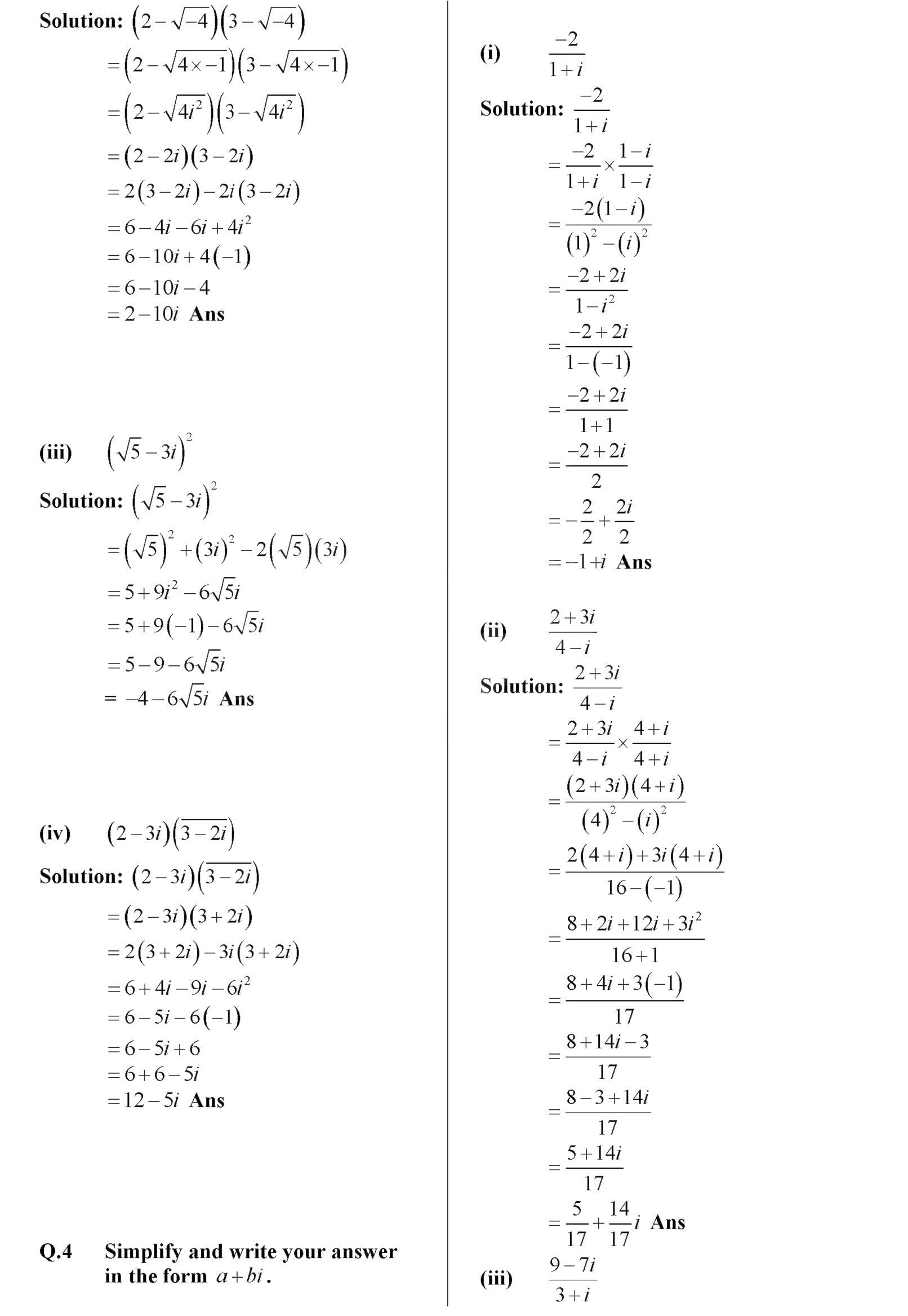# 9th class Math solved Notes chapter: Real and Complex Numbers{ Exercise 2.6}9th class Math solved Notes chapter: Real and Complex Numbers{ Exercise 2.6}

9th class Math solved Notes chapter: Real and Complex Numbers{ Exercise 2.6}

9th class Math solved Notes chapter: Real and Complex Numbers{ Exercise 2.6}

9th class Math solved Notes chapter: Real and Complex Numbers{ Exercise 2.6}